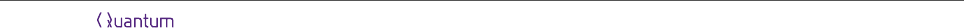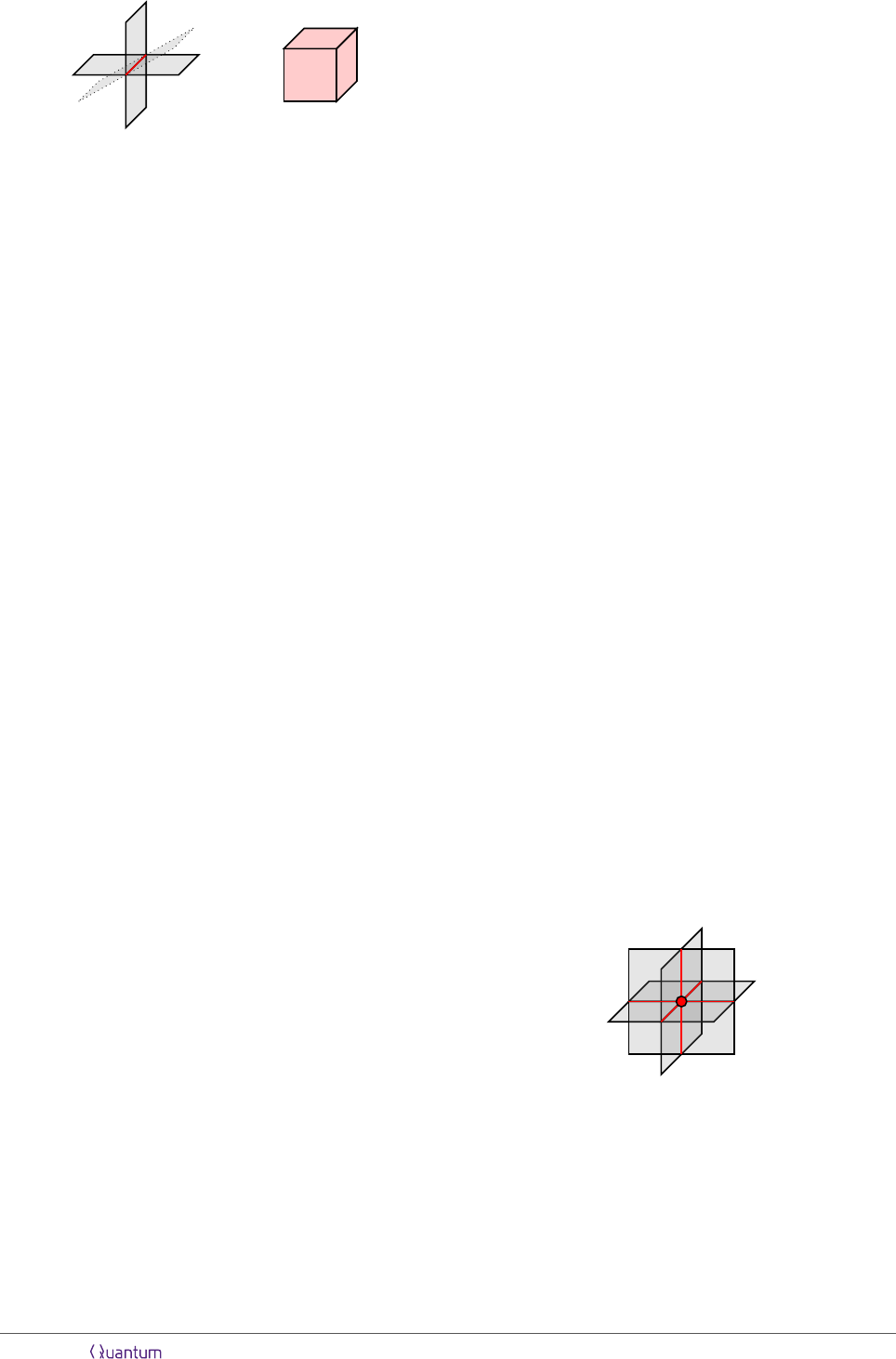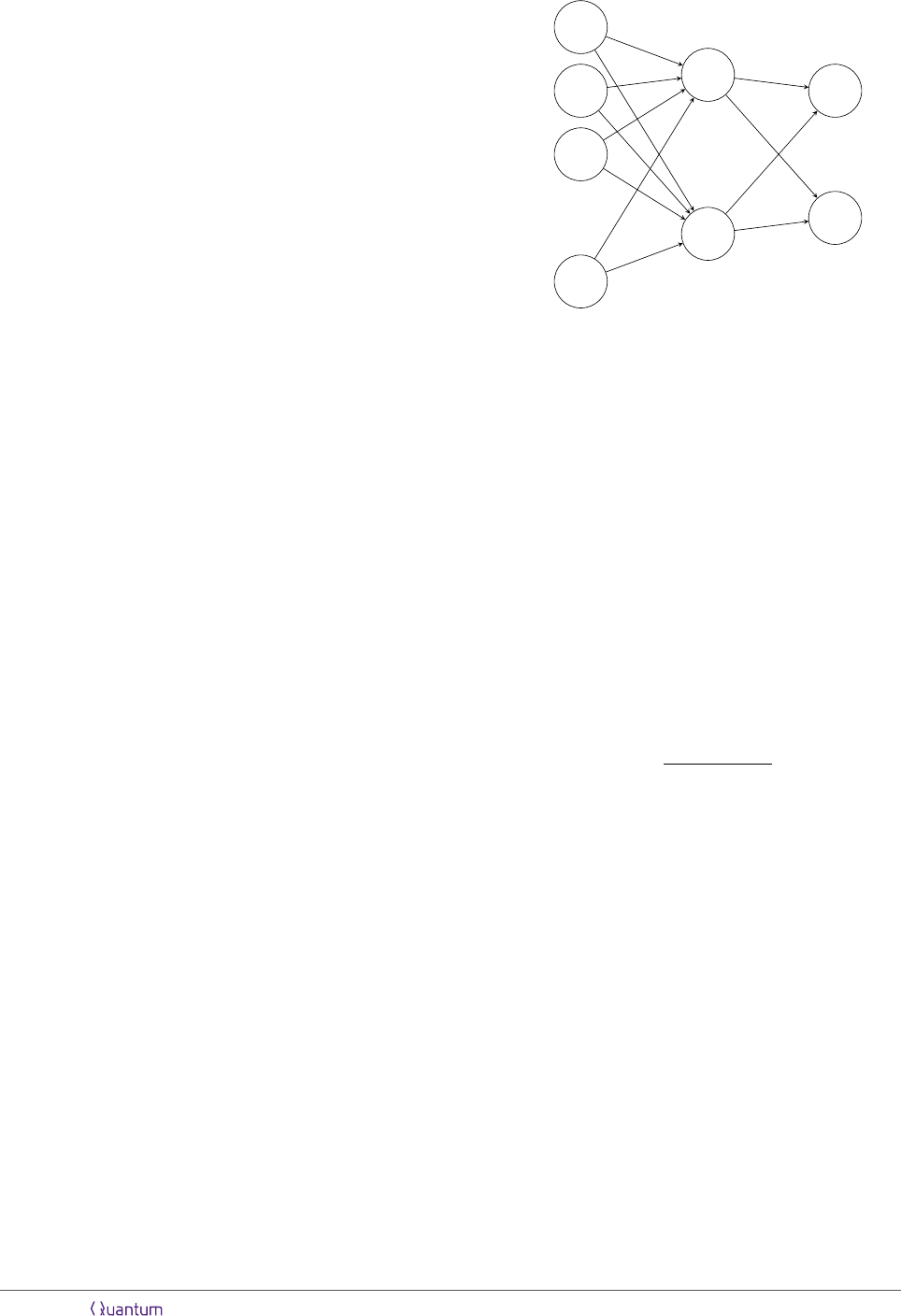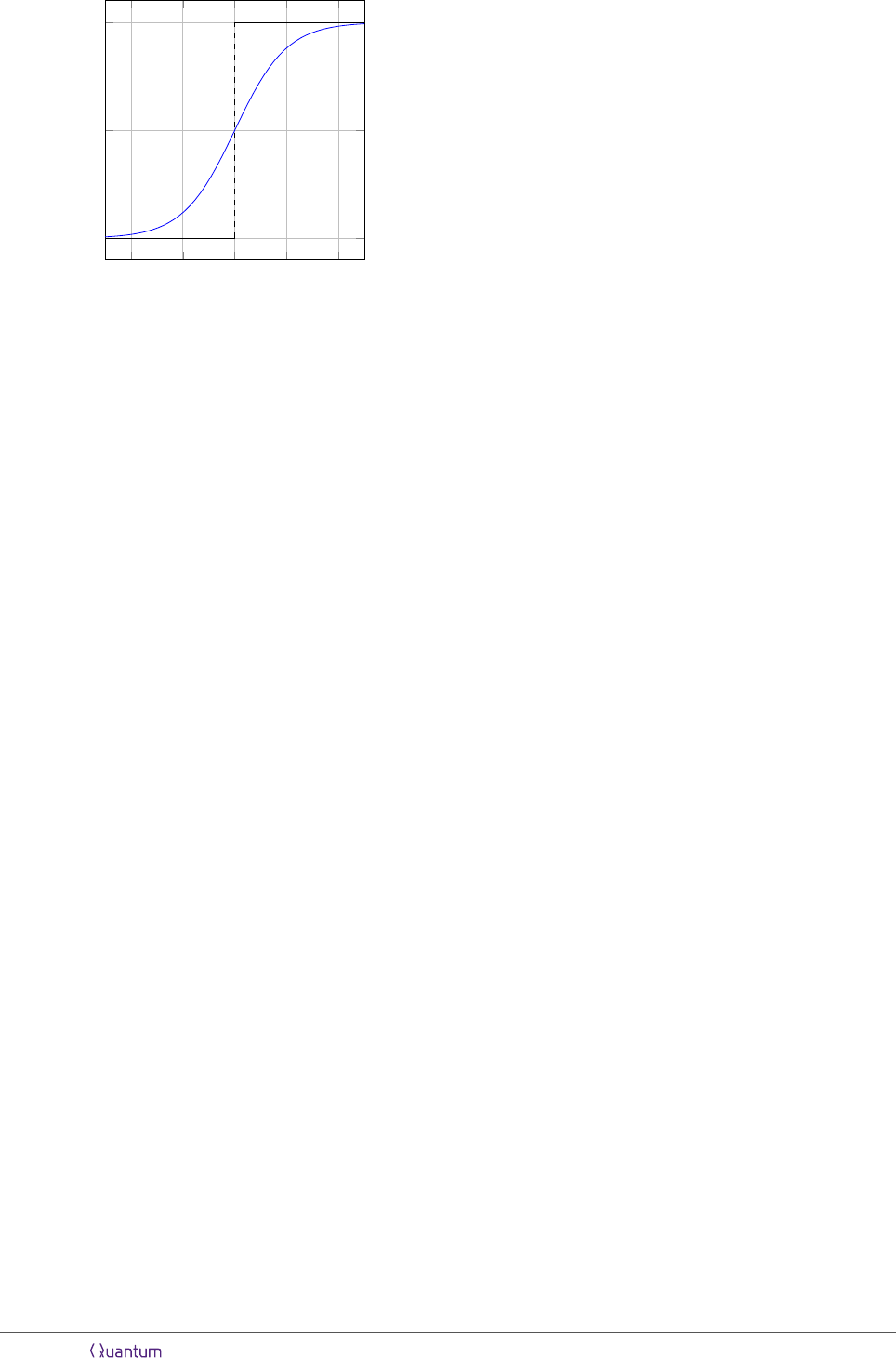Scalable Neural Network Decoders
for Higher Dimensional Quantum Codes
N. P. Breuckmann
1
and X. Ni
1,2
1
Institute for Quantum Information, RWTH Aachen University, Germany
2
Max Planck Institute of Quantum Optics, Germany
May 16, 2018
Machine learning has the potential to
become an important tool in quantum er-
ror correction as it allows the decoder
to adapt to the error distribution of a
for using neural networks is the fact that
they can be evaluated by dedicated hard-
ware which is very fast and consumes little
power. Machine learning has been previ-
ously applied to decode the surface code.
However, these approaches are not scal-
able as the training has to be redone for
every system size which becomes increas-
ingly diﬃcult. In this work the existence
of local decoders for higher dimensional
codes leads us to use a low-depth convo-
lutional neural network to locally assign
a likelihood of error on each qubit. For
noiseless syndrome measurements, numer-
ical simulations show that the decoder has
a threshold of around 7.1% when applied
to the 4D toric code. When the syndrome
measurements are noisy, the decoder per-
forms better for larger code sizes when
the error probability is low. We also give
theoretical and numerical analysis to show
how a convolutional neural network is dif-
ferent from the 1-nearest neighbor algo-
rithm, which is a baseline machine learning
method.
1 Introduction
A full-featured quantum computer will rely on
some form of error correction as physical qubits
are prone to the eﬀects of environmental noise.
The authors contribute equally to this work.
When using error correcting codes, decoders play
a large role in the performance of the fault-
tolerant protocols. Using neural networks to de-
code the surface code has been suggested in ear-
lier works [3, 21, 36, 37]. The primary motivation
inspiring these works to use neural networks is
their ability to adapt to the error model. How-
ever, an issue that has not been addressed so far
is scalability: in previous approaches, the neu-
ral networks have to be re-trained for every sys-
tem size, despite the fact that in general machine
learning methods become problematic when the
input space becomes too large. Thus, it is in-
teresting to ask whether there exists a family of
quantum error correcting codes, which we can de-
code using neural networks in a clearly scalable
way.
To provide a positive answer to this question,
we introduce a decoder for the four-dimensional
version of the toric code based on neural net-
works for which the training only has to be per-
formed once on a small system size. Afterwards
the decoder can be scaled up to arbitrary system
sizes without re-training. The layout of the net-
work is a convolutional neural network which are
widely used as a building block in image recogni-
tion. Furthermore, the neural network is con-
stant depth with respect to the scaling of the
system size. Our approach is informed by the
existence of local decoders for the 4D toric code
[6, 7, 11, 28]. A drawback of those decoders
is their low threshold of less than 2% against
independent noise (assuming perfect syndrome
measurements). The (global) minimum-weight
decoder, on the other hand, has a threshold of
10.1%  but it is not known to be computa-
tionally eﬃcient. Our decoder shown a threshold
of 7.1% while having a complexity close to local
decoders. This result is very close to the 7.3%
Accepted in Quantum 2018-05-04, click title to verify 1
arXiv:1710.09489v3 [quant-ph] 14 May 2018reported in  which uses a renormalization ap-
proach. When the syndrome measurements are
noisy, our decoder still performs better for larger
lattice when error probability is low, see Fig-
ure 10 for the plot. More numerical simulation
needs to be done to determine the threshold.
This paper is structured as follows. In sec-
tion 2 we discuss previous work in which ma-
chine learning was applied to decode quantum
codes. In section 3 we give a review of the 4D
toric code and its properties. In section 4, we
provide a general introduction to machine learn-
ing. In section 5 we describe the application of
convolutional neural networks as a subroutine in
our decoding algorithm. In section 6 we discuss
the results of Monte-Carlo simulations. In Ap-
pendix A, we give a short introduction to the
backpropagation algorithm which we use to train
the neural network. In Appendix D, we give a
toy example which shows how a baseline machine
learning model can fail at a task similar to de-
coding when the system size becomes large. This
highlights the importance of choosing a transla-
tional invariant model as we do. In Appendix E,
we observed and analyzed one property of multi-
layer convolutional neural networks, and show it
ﬁts nicely to the task of assigning likelihood of
qubit errors for high dimensional toric code.
2 Previous Work
It is known for a while in the classical error cor-
rection community that the errors and syndromes
of a parity checking code can be put together into
a probabilistic graphical model, which is a major
tool for machine learning. Probabilistic graphical
models include for example Bayesian networks
and the Ising model (see  for an introduction
to probabilistic graphical model and connection
to error correcting codes). In  (and references
therein), it is shown that by using belief propa-
gation, the decoder can achieve very good perfor-
mance for LDPC and turbo code that are close to
the Shannon limit. We want to note that while
there is no formal connection (to our knowledge)
between neural networks and graphical models, a
graphical model of a problem is often helpful for
designing the architecture of the neural network.
For example, in  the structure of the Tanner
graph is used to construct a neural network, in
order to improve belief propagation for (classical)
codes that have high density checks.
In , the authors discussed applying be-
lief propagation to decoding of quantum stabi-
lizer codes. While stabilizer codes and classi-
cal parity checking codes share many similarities,
the authors highlighted several issues that might
cause belief propagation to work much worse for
stabilizer codes (even with low density checks).
In [12, 13], the authors used belief propagation as
a subroutine in the renormalization decoder for
topological codes, with the purpose of synchro-
nizing marginal probabilities across neighbouring
cells.
Neural network decoder for quantum stabilizer
codes has been studied in [3, 21, 36, 37] (more
precisely in  hidden Boltzmann machines are
used instead of neural networks). Our work will
use a similar scheme as [21, 36], where the neural
networks predict which qubits have undergone an
error. In [3, 37], the neural networks output 1 or
2 bits which will correct the ﬁnal measurement of
the logical operators. The main diﬀerence of our
work is that our machine learning decoder is nat-
urally scalable through the use of convolutional
neural networks.
3 The 4D Toric Code
3.1 Deﬁnition
The 4D toric code is a stabilizer quantum code
deﬁned on a four-dimensional (4D) hypercubic
lattice which was ﬁrst considered in . We will
consider periodic boundary conditions so that
topologically the lattice is a 4D torus. The faces
of the lattice are squares and they are identi-
ﬁed with the qubits. From now on we will use
the words face and qubit interchangeably. The
number of faces in a lattice of side-length L is
4
2
L
4
= 6L
4
. This follows from the fact that
every face is uniquely determined by a vertex v
and two coordinates i, j {x, y, z, w}, so that the
face with base-point v lies in the i-j-plane. More
generally, the number of k-dimensional objects
in the lattice (1-dimensional objects would be
edges or 3-dimensional objects would be cubes)
is given by
4
k
L
4
. There are stabilizer checks for
every edge and cube in the lattice. A stabilizer
check associated with a particular edge acts as
Pauli-X on all faces which are incident to it (see
Figure 1 (left)), whereas a stabilizer check associ-
ated with a particular cube acts as Pauli-Z on all
Accepted in Quantum 2018-05-04, click title to verify 2Figure 1: The stabilizer checks of the 4D toric code
correspond to edges which act as Pauli-X on all qubits
incident to an edge (left) and cubes which act as Pauli-Z
on all qubits incident to an edge (right).
faces incident to this particular cube (see Figure 1
(right)). All stabilizer checks act on 6 qubits and
each qubit is acted upon by 8 stabilizer checks.
Consider an operator E acting as Pauli-Z on
some subset of qubits. For E to commute with
an X-check their overlap has to be even. Hence,
to commute with all X-checks E can not contain
any connected components that have a bound-
ary where a single face is incident to an edge.
Geometrically this means that E has to be a col-
lection of boundaryless (closed) surfaces. If E
itself is the boundary of a 3D volume inside the
hypercubic lattice then it is the product of all Z-
stabilizer elements inside this volume. But there
are other examples of surfaces which do not have
a boundary: Since the topology of the lattice
is non-trivial we can consider a sheet extending
over the whole x-y-plane. Due to the periodic-
ity of the lattice this sheet has no boundary, but
is not itself the boundary of any 3D volume and
thus it is not the product of any Z-stabilizers.
It is therefore a logical operator. There is one
such operator for every plane in the lattice. Each
plane is labelled by two coordinates (x-y-plane,
x-z-plane, . . . ) so that the 4D toric code en-
codes
4
2
= 6 logical qubits. A sheet extending
through the whole lattice consists of at least L
2
faces which means that the 4D toric code has dis-
tance growing quadratically with the number of
physical qubits. The parameters of the code are
[[n = 6L
4
, k = 6, d = L
2
]].
In  the authors argue that the threshold
of the 4D toric code against independent bit-ﬂip
and phase-ﬂip errors is approximately p
c
0.11
under minimum-weight decoding. This is the
same threshold as for the 2D toric code and the
surface code.
3.2 Syndromes
A feature of the 4D toric code is that the set of
violated stabilizer checks form extended objects:
since we pick up the boundary of an error (which
is a collection of surfaces), the syndrome will al-
ways form closed loops as opposed to a set of
points for the 2D toric code or surface code. A
minimum-weight decoding, similar to minimum-
weight perfect matching for the 2D toric code, is
ﬁnding a minimum-area surface among all sur-
faces that have the syndrome as its boundary.
It is known that the problem of ﬁnding such
a minimum-are surface can be solved eﬃciently
when the lattice is three-dimensional , but it
is open whether it can be solved eﬃciently for a
4D lattice. However, there are several decoders
with worse error-correction performance than the
minimum weight decoder but which are compu-
tationally eﬃcient [1, 7, 11, 18, 28]. The com-
mon way these decoders operate is by iteratively
shortening the syndrome loops by ﬂipping nearby
faces.
The fact that the syndromes form closed loops
can be understood in terms of local linear depen-
dencies between the stabilizer elements: Taking
all X-checks (edges) which are incident to a par-
ticular vertex gives the identity operator. This is
the case because for every triple of vertex, edge
and face which are pairwise incident to one an-
other we can always ﬁnd one more edge which
is incident to the same vertex and face (see Fig-
ure 2). Similarly, taking all Z-checks (cubes) of
one hypercube gives the identity since there are
two cubes are overlapping on every face of the
hypercube.
Figure 2: The dependency of the edge stabilizers which
are incident to a common vertex. Taking the product
of all edge-stabilizers (red) incident to a common vertex
(red) gives the identity.
Each of those local linear dependencies can
be interpreted as a (classical) parity check on
the syndrome. More explicitly: Both the X-
Accepted in Quantum 2018-05-04, click title to verify 3syndrome and the Z-syndrome are encoded by
a classical linear code. The code words of this
code are the valid syndromes. What are the pa-
rameters of this code? - There is a bit for every
X-check (edge) in the lattice, so the block size
of the code is 4L
4
. There is a local dependency
for every vertex. However, the local dependen-
cies themselves are not independent. Taking the
product over all vertices and all edge-checks in-
cident to this vertex gives the identity since ev-
ery edge is incident to two vertices. Hence, the
number of independent checks in the classical lin-
ear code encoding the syndrome information is
L
4
1. The encoded syndrome information there-
fore contains 4L
4
(L
4
1) = 3L
4
+ 1 bits. The
distance of the classical code is 4 since adding
the boundary of a face takes us from one valid
syndrome to another valid syndrome.
Since the syndrome of the 4D toric code is
encoded it has some build-in robustness against
syndrome errors. In comparison, it is known
that the 2D toric code does not have a decoding
threshold in the presence of syndrome noise. The
parity measurements have to be repeated and the
record of the repeated syndrome measurements
is decoded. This essentially implements a repe-
tition code in time. Repeating measurements is
not necessary for the 4D toric code to have in-
creased error suppression capabilities with larger
system size. The fact that measurements are not
repeated is referred to as single-shot measure-
ments. It has been shown analytically in  that
a single-shot decoder can have a threshold and
single-shot decoders for the 4D toric code have
analyzed numerically in [2, 7, 14].
As 4D space is hard to visualize it is useful
to consider the 3D toric code to gain some geo-
metric intuition. It is deﬁned on a 3D cubic lat-
tice with periodic boundaries. Similarly to the
4D toric code the qubits are identiﬁed with the
faces of the lattice and the X-stabilizer checks
act on all faces incident to an edge (weight 4)
while the Z-stabilizer checks act on all faces inci-
dent to a cube (weight 6). A boundaryless sheet
acting as Pauli-Z commutes with all X-stabilizer
checks just as for the 4D toric code. The X-
stabilizer checks also satisfy the local linear de-
pendencies shown in Figure 2. To commute with
all Z-stabilizer checks it suﬃces to take a closed
loop of Pauli-X operators in the dual lattice. The
existence of these string-like logical operators re-
sults in a threshold which is generally lower under
minimum-weight decoding [33, 38].
4 Basics of Machine Learning
To have a better understanding of our decod-
ing procedure, it is useful to ﬁrst give a small
overview of machine learning. This will provide
insights to advantages and limitations of our pro-
cedure, and possible ways to improve it. For more
extensive review on machine learning and in par-
ticular neural networks, we refer to [17, 22, 26].
Machine learning is often categorized into su-
pervised learning, unsupervised learning and re-
inforcement learning, each has a diﬀerent focus.
However, all of them usually involve the use of
some models. In this paper, the word “model”
simply means a family of functions f
α
or sub-
routines s
α
, where the (most likely multidimen-
sional) parameter α needs to be trained by cer-
tain learning algorithms. Below we give a not
very comprehensive introduction to these three
kinds of learning
Supervised learning is to ﬁnd α such that
f
α
= f
hidden
, where f
hidden
is given im-
plicitly by a dataset of input-output pairs
(x
i
, y
i
= f
hidden
(x
i
)).
Unsupervised learning refers to many diﬀer-
ent tasks which are related to ﬁnding struc-
tures in a dataset. In contrary to supervised
learning, there is no desired output for each
entry in the dataset. For example, consider-
ing tasks in error correction, inferring prop-
erties of the error model from a dataset of
syndromes or using the dataset to generate
new similar syndromes can be viewed as un-
supervised learning, as well as some tasks
studied in .
Reinforcement learning is concerned with
how agents ought to take actions in an en-
vironment so as to maximize some notion of
cumulative reward. However, in this paper
we will use the word “reinforcement learn-
ing” to denote the optimization of a subrou-
tine s
α
of a program, so that at the end of the
program a predeﬁned score is maximized.
For complicated tasks, it is beneﬁcial to com-
bine these approaches. A notable example which
Accepted in Quantum 2018-05-04, click title to verify 4highlights this is the recent success of the AI Al-
phaGo [30, 31]. We give a short summary of this
combined approach in Appendix C and discuss
some ideas for applying it to the decoding prob-
lem of quantum codes.
One major diﬃculty encountered in machine
learning is the overwhelmingly large input/state
space. Here we will only give a short explanation
of how this diﬃculty arises in supervised learn-
ing. Recall that we are given a dataset (x
i
, y
i
)
with the goal being approximating f
hidden
. If the
input space is large, then for a random x, it is
unlikely that x is “similar” to any of the x
i
from
the dataset. Therefore, it is hard to guess the
value of f
hidden
(x) and approximate it by f
α
(x).
This is why often the most important part of a
machine learning project is to choose a suitable
model, usually by encoding the prior knowledge
of the task into it. The model we use in this pa-
per is neural networks which we introduce in the
following section.
4.1 Basics of Neural Networks
A neural network is a directed, multipartite
graph consisting of layers l = 0, . . . , L. The ver-
tices in layer l are connected to vertices in the
following layer l + 1.
The vertices of the network are called neu-
rons. The main idea behind the neural network
is the following: Each neuron computes a primi-
tive non-linear function f
w,b
: R
q
R, for which
the input values are given by neurons of the
previous layer connected to it. The subscripts
w R
q
and b R are parameters which can dif-
fer for each neuron. Before we discuss the func-
tion f
w,b
in more detail let us ﬁrst understand
how the network performs a computation. The
neurons in the ﬁrst layer do not have any pre-
decessors and their output is simply set to be
the input of the network, which is a bit string
x {0, 1}
m
. The values of the neurons in the
last layer are interpreted as the output of the
network. We see that the network describes a
function F : {0, 1}
m
[0, 1]
n
. The ﬁrst layer
l = 0 is called the input layer and the last layer
l = L is called the output layer. All other layers
l = 1, . . . , L1 are called hidden layers since they
are considered to be internal to the network.
The parameters w and b are called weights and
biases. They deﬁne a linear map w · y + b where
y is the input of the neuron. The function that
.
.
.
.
.
.
.
.
.
x
1
x
2
x
3
x
m
F
N
1
(x)
F
N
n
(x)
Input layer
l = 0
Hidden layer
l = 1
Ouput layer
l = 2
Figure 3: A neural network consisting of 3 layers. The
network takes input x {0, 1}
m
which is represented
as the ﬁrst layer of neurons. The values of neurons in
the hidden layer and the output layer are given by the
function f
w,b
: R
q
[0, 1] evaluated on their input
(indicated by q incoming arrows). The parameters w
R
q
and b R, called weights and bias, can be diﬀerent
for each neuron. The values of the neurons in the last
layer are the output of the network F
N
(x) R
n
.
each neuron computes has the form:
f
w,b
: R
q
[0, 1], y 7→ σ(w · y + b) (1)
where in this paper σ is either tanh or the sigmoid
function
σ(z) =
1
1 + exp(z)
(2)
which is plotted in Figure 4. Both non-linear
functions can be thought of as a smoothed step
function. The smoothness of σ is important to
the training of the neural networks as it is based
on optimizing certain objective function with gra-
dient descent.
Let us have a look at a small example which
gives some intuition why neural networks are able
to perform interesting computations: Consider a
single neuron which takes q = 2 inputs and has
weights w = (12, 12) and bias b = 17. The
neuron computes the following values for each in-
put:
f(0, 0) = σ(17) 1
f(1, 0) = σ(5) 1
f(0, 1) = σ(5) 1
f(1, 1) = σ(7) 0.
(3)
Accepted in Quantum 2018-05-04, click title to verify 54 2 0 2 4
0
0.5
1
σ(z)
Θ(z)
Figure 4: The sigmoid function σ is a smooth version of
the Heaviside step function Θ.
We observe that these are approximately the in-
put/output relations of the NAND gate. The
approximation can be made arbitrarily close by
increasing the absolute values of the weights w
and the bias b. Since any Boolean function
F : {0, 1}
m
{0, 1}
n
can be computed by a
network of NAND gates, there consequently also
exists a representation of F as a neural network.
However, in practice it is more eﬃcient to adapt
the connectivity of the network to the problem
at hand.
The process of training the network, i.e. grad-
ually adapting the values of the weights w and
biases b to make the network a desired function,
is described in Appendix A.
4.2 Convolutional Neural Networks
Compared to fully connected neural networks,
convolutional neural networks (CNN) require
much fewer parameters to describe. We will start
with the deﬁnition of one convolutional layer.
The input to the layer resides on a D-dimensional
lattice of size L
D
. On each lattice site, there is
a d-dimensional vector x
u
R
d
, where the sub-
script u Z
D
L
(we use Z
L
to denote integer in
range [0, L 1]). We deﬁne the kernel to be a
vector K
u,i
, where u Z
D
n
and i Z
d
. With a
slight abuse of notation, we will say such a kernel
has size n
D
. The convolution is then
y
v
=
X
uZ
D
n
X
iZ
d
x
vu,i
K
u,i
, (4)
where x
vu,i
is the ith element of x
vu
, and each
element of v has range [n1, L1]. The index v
of the output y can be shifted according to needs
(e.g. to Z
D
Ln+1
). In this paper, we solely work
with input that has the periodic boundary con-
dition. Thus, we can indeed treat the indices u